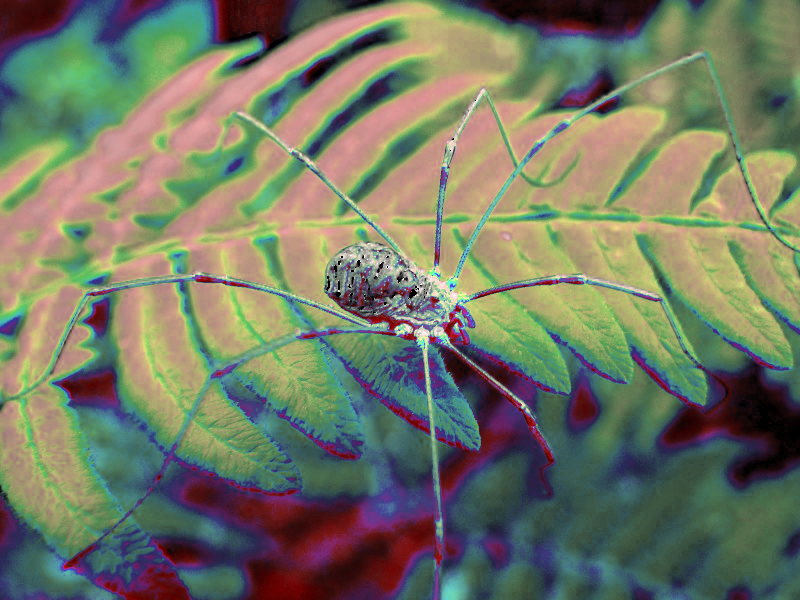Performs an HSV adjustment operation on a pixmap and returns the result.

## Syntax

• `AdjustHSV(pm, A)`

• `pm` is a pixmap

• `A` is a matrix

## Description

If `pm` is a pixmap and `A` an HSV adjustment matrix, then `AdjustHSV(pm, A)` returns the pixmap obtained by applying `A` to `pm`.

An HSV adjustment matrix is a real 3×5 matrix of the form

```    ⎛ hc   hh   hs   hv   ho   ⎞
A = ⎜ sc   sh   ss   sv   so   ⎟
⎝ vc   vh   vs   vv   vo   ⎠```

Each pixel in `pm` is transformed in the same way. If a pixel has colour `hsv(h, s, v)`, then its new HSV coordinates are obtained first by multiplying the four first columns of `A` by the vector ❨1, h, s, v❩:

```                              ⎛1⎞
⎛h'⎞   ⎛ hc   hh   hs   hv   ⎞⎜h⎟
⎜s'⎟ = ⎜ sc   sh   ss   sv   ⎟⎜s⎟
⎝v'⎠   ⎝ vc   vh   vs   vv   ⎠⎝v⎠.```

The final column of `A` decides how the vector ❨h', s', v'❩ is made into a valid HSV coordinate vector, in which each component is restricted to `[0, 360]` (for h) or `[0, 1]` (for s, v). If `ho` is `0`, then `h'` is moved into `[0, 360]` by clamping; otherwise, `h'` is moved into `[0, 360]` by wrapping. The saturation and value coordinates are treated similarly depending on `so` and `vo`, respectively.

Notice that this function can be used to shift the hue, alter the saturation and value, and to mix HSV channels.

## Examples

`A ≔ ❨❨180, 0, 360, −360, 0❩, ❨0, 0, 1, 0, 0❩, ❨.5, −.005, 0, 1, 0❩❩`
```⎛   180       0     360    −360       0⎞
⎜     0       0       1       0       0⎟
⎝   0.5  −0.005       0       1       0⎠
```
`AdjustHSV(ExampleData("harvestman"), A)`